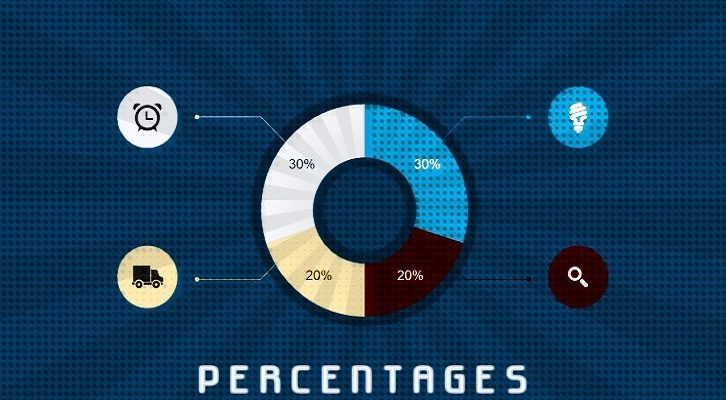# CAT – Percentages

This is an interesting question from Percentages. Simple framework, but finding the answer is not that easy

Question
A is x% more than B and is x% of sum of A and B. What is the value of x? Give approximate answer.

Roughly 62%

a = b (1 + x) => a/b = 1 + x

a = x (a + b) , dividing by a through out
1 = x (1 + b/a)
1 = x (1 + 1/(1 + x))
1 = x (x + 2) / (x + 1)
x + 1 = x2 + 2x
=> x2 + x – 1 = 0
Now, we need to solve this equation. Using the discriminant method, when we solve this, x turns out to be {-1 + sqrt (5)}/2
x has to lie between 0 and 1 and therefor cannot be { -1 – sqrt (5) }/2.
So, the only solution is { -1 + sqrt (5) }/2. This is roughly 0.62.
Or, x has to be 62% approximately. The ration 1.618 is also called the golden ratio, and is the conjugate and reciprocal of 0.618.
The golden ratio finds many mentions, from the Fibonacci series to Da Vinci. So, it is a big favourite of mathematician.